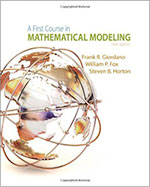A First Course in Mathematical Modeling, 5th Edition - Maplesoft Books - Maple Books, Maple Resources and Math BooksHome : Maplesoft Books : A First Course in Mathematical Modeling, 5th EditionDetails Search# A First Course in Mathematical Modeling, 5th Edition

## 2013

 Tell others about this book: Tweet
Description
Offering a solid introduction to the entire modeling process, A FIRST COURSE IN MATHEMATICAL MODELING, 5th Edition delivers a balance of theory and practice, and gives relevant, hands-on experience developing and sharpening modeling skills. Throughout, the book emphasizes key facets of modeling, including creative and empirical model construction, model analysis, and model research, and provides myriad opportunities for practice. The authors apply a six-step process to enhance problem-solving capabilities, whatever the reader's level. In addition, rather than simply emphasizing the calculation step, the authors first help the reader learn how to identify problems, construct or select models, and figure out what data needs to be collected. By involving the reader in the mathematical process as early as possible -- beginning with short projects -- this text facilitates progressive development in mathematics and modeling. Maple is used to enhance discussions related to difference equations, proportionality, model fitting (least squares), empirical models, simulation, linear programming, dimensional analysis, modeling with differential equations, modeling with systems of differential equations, and optimization of continuous models.
Categories:
Other Details
Language: English
ISBN: 978-1285050904
Publisher: Brooks Cole
 Do you know of a book that should be included in our list? Tell us about it!
 Title/Author: Category: Any Category Abstract Algebra Biology Calculus Chemistry Complex Analysis Computer Algebra Computer Science Conference Proceedings Courseware Cryptography Differential Equations Differential Geometry Dynamical Systems Economics Engineering Games Linear Algebra Maple Manuals Mathematical Modeling Number Theory Numerical Analysis Operations Research Optimization Physics Pre-Calculus Probability & Statistics Programming Recent Releases Vector Calculus Language: Any Language English French German Spanish Japanese Dutch Russian Swedish Polish Persian ChineseAbstract Algebra Biology Calculus Chemistry Complex Analysis Computer Algebra Computer Science Conference Proceedings Courseware Cryptography Differential Equations Differential Geometry Dynamical Systems Economics Engineering Games Linear Algebra Maple Manuals Mathematical Modeling Number Theory Numerical Analysis Operations Research Optimization Physics Pre-Calculus Probability & Statistics Programming Recent Releases Vector Calculus